# Interval dimension of a partially ordered set

(diff) ← Older revision | Latest revision (diff) | Newer revision → (diff)

Letbe a partially ordered set. Its interval dimension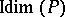is the leastsuch that there are interval extensions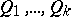ofsuch that for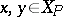one hasexactly if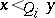for all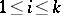. An interval extension ofis an extension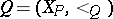of(i.e.,implies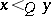), such thatis an interval order. Since every linear extension ofis an interval extension, the interval dimension is related to the dimension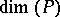(cf. Dimension of a partially ordered set) by the inequality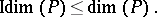For partially ordered setsand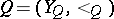one says thathas an interval representation onif there are mappingsand, such that

1)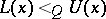, i.e,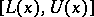is a non-degenerate interval offor each;

2)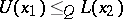exactly if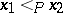. The inequality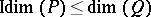holds for any two partially ordered setsandsuch thathas an interval representation on. There are partially ordered setswith the property thathas an interval representation onand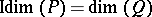. For the most notable construction of such a, define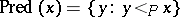,and. Let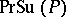denote the inclusion ordering on the set. It can be shown thathas an interval representation on, that, and that in some senseis the smallest partially ordered set admitting an interval representation of, see [a3].

The concept lattice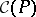is the lattice completion of. Thereforeadmits an interval representation of, and again. It can be shown that every latticesuch thathas an interval representation oncontainsas suborder, see [a5].

The mapping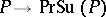can be used to transform questions about interval dimensions of partially ordered sets into questions about dimensions. Two instances of this approach are given below.

### Recognizing partially ordered sets of interval dimension two.

This problem can be reduced to recognition of partially ordered sets of dimension two. The bottleneck of the obvious approach is the construction of. The fastest reduction [a4] avoids this step and has time complexity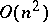. The decision problem for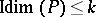is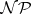-complete for every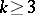(cf. also Complexity theory).

### Characterization of-interval irreducible partially ordered sets.

A-interval irreducible partially ordered set is a partially ordered set of interval dimension three whose interval dimension drops to two upon deletion of any element. This characterization problem was attacked in two steps. W.T. Trotter [a6] gave a complete list of bipartite-interval irreducible partially ordered sets. S. Felsner [a3] proved that every-interval irreducible partially ordered set is the reduced partial stack of a partially ordered set in Trotter's list and that a complete list of-interval irreducible partially ordered set is too complex to be given explicitly. In particular, every two-dimensional partially ordered set is a suborder of some-interval irreducible partially ordered set. Both parts of this work depend deeply on the list of-irreducible partially ordered sets for ordinary dimension.

### Tractability.

In some cases, interval dimension is more tractable than dimension. Compare, for example, the forbidden-suborder characterization of the inequality, whereis an anti-chain for ordinary dimension with the result for interval dimension.

Another example is the positive solution to the removable-pair problem. For ordinary dimension it is conjectured that every partially ordered set of at least three points contains a pair of points whose removal decreases the dimension by at most one. This conjecture remains one of the most tantalizing open problems in the field (1998). However, the interval dimension is reduced by at most one upon removal of any critical pair. The proof of this can be given by a straightforward construction.

There is also a beautiful connection with graph dimension, see [a2] and the references therein. For a graph, let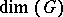be the leastsuch that there are linear ordersonsuch that for every edge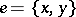and every vertexthere is an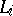with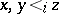. The incidence poset ofis the partially ordered set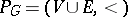, where the order relation takes a vertexbelow an edgeif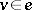. It can be shown that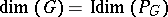.

How to Cite This Entry:
Interval dimension of a partially ordered set. Encyclopedia of Mathematics. URL: http://encyclopediaofmath.org/index.php?title=Interval_dimension_of_a_partially_ordered_set&oldid=17636
This article was adapted from an original article by S. Felsner (originator), which appeared in Encyclopedia of Mathematics - ISBN 1402006098. See original article